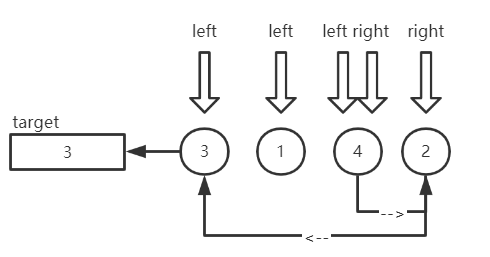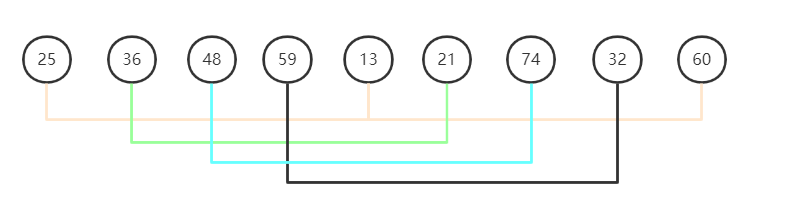# 前言

 交换排序 冒泡排序（Bubble Sort） 快速排序（Quick Sort） 插入排序 简单插入排序（Simple Insertion Sort）（也被称为直接插入排序） 希尔排序（Shell Sort） 选择排序 简单选择排序（Simple Selection Sort）（也被称为直接选择排序） 堆排序（Heap Sort） 归并排序 Merge Sort 基数排序 Radix Sort 计数排序 Count Sort

| 算法名称 | 时间复杂度 | 空间复杂度 | 稳定性 | 复杂性 |
|:----😐:----😐:----😐:----😐:----😐:----😐:----😐:----😐
| 冒泡排序 | O(n2) | O(1) | 稳定 | 简单 |
| 快速排序 | O(nlog2n) | O(log2n) | 不稳定 | 较复杂 |
| 简单插入排序 | O(n2) | O(1) | 稳定 | 简单 |
| 希尔排序 | O(nlog2n)（参考值，与增量有关） | O(1) | 不稳定 | 较复杂 |
| 简单选择排序 | O(n2) | O(1) | 不稳定 | 简单 |
| 堆排序 | O(nlog2n) | O(1) | 不稳定 | 较复杂 |
| 归并排序 | O(nlog2n) | O(n) | 稳定 | 较复杂 |
| 基数排序 | O(d(n + r)) | O(n + r) | 稳定 | 较复杂 |
| 计数排序 | O(n + k) | O(n + k) | 稳定 | 简单 |

## 说明

• 排序还会涉及到一个概念，叫排序的稳定性。那么稳定性是什么意思呢？
稳定性，就是有两个相同的元素，排序后它们相对位置是否发生变化，若未变化则该称该排序算法是稳定的。否则就是不稳定的。
• 本次总结所有的算法实现均是以从小到大为例进行排序的，后面不再特别指出。
• 文章后面将会提到的有序区，无序区。是指在待排序列中，已经排好顺序的元素，就认为其是处于有序区中，还没有被排序的元素就处于无序区中。
• 小提示，点击文章页面右下角的“查看目录”可以查看本文目录哦

# 交换排序

## 冒泡排序

### 复杂度与稳定性与优缺点

• 空间复杂度：O(1)
• 时间复杂度：O(n2)
• 最好情况：正序有序时，普通冒泡排序仍是O(n^2)，优化后的冒泡排序是O(n)
• 最坏情况：逆序有序时，O(n2)
• 稳定性：稳定
• 优点：简单，稳定，空间复杂度低
• 缺点：时间复杂度高，效率不好，每次只能移动相邻两个元素，比较次数多

### 算法实现

public void BubbleSort(int[] array){
for(int i = 0; i < array.Length - 1; i ++){
for(int j = array.Length - 1; j > i ; j --){
if(array[j] < array[j - 1]){
Swap(array, j, j - 1);
}
}
}
}

// 实现将指定下标的两个元素在数组中交换位置
public void Swap(int[] array, int i, int j){
int temp = array[i];
array[i] = array[j];
array[j] = temp;
}


【算法解读】

【举个栗子】

### 冒泡排序优化

public void BubbleSortOptimize(int[] array){
for(int i = 0; i < array.Length - 1; i ++){
bool swapTag = false; // 标志位，判断每完成一趟冒牌排序是否发生交换
for(int j = array.Length - 1; j > i; j --){
if(array[j] < array[j - 1]){
Swap(array, j, j -1);
swapTag = true;
}
}
if(!swapTag){
break;
}
}
}


## 快速排序

### 复杂度与稳定性与优缺点

• 空间复杂度：O(log2n)取决于递归深度，所占用的栈空间
• 时间复杂度：O(nlog2n)
• 最好情况：O(nlog2n)，当每次分区，分界点左，右两边的分组长度大致相等时，快速排序算法的性能最好
• 最坏情况：O(n2)，当每次选择的分界点都是当前分组的最大元素或最小元素时，快速排序算法退化为冒泡算法。
• 稳定性：不稳定，因为将元素移动到分界点两边时，会打乱原本相等元素的顺序
• 优点：极快
• 缺点：不稳定

### 算法实现

public void QuickSort(int[] array){
QuickSortImpl(array, 0, array.Length - 1);
}

public void QuickSortImpl(int[] array, int left, int right){
if(left >= right) return;
int pivot = Partition(array, left, right);
QuickSortImpl(array, left, pivot - 1);
QuickSortImpl(array, pivot + 1, right);
}

// 分区函数，实现将所有元素依据大小移动到分界点的两边
public int Partition(int[] array, int left, int right){
int target = array[left];
while(left < right){
while(right > left && array[right] >= target){
right --;
}
if(right > left){
array[left] = array[right];
// 此时left位置的元素是被移动过来的元素，肯定比目标元素小
// 所以左指针扫描时就可以不用比较该元素，进行left++操作
left ++;
}
while(left < right && array[left] <= target){
left ++;
}
if(left < right){
array[right] = array[left];
right --;
}
}
array[left] = target;
return left;
}


【算法解读】

【举个栗子】### 快速排序优化策略

• 当待排序列是部分有序时，固定选取枢轴（即分界点）会使快排效率低下，要缓解这种情况，可以引入随机选取枢轴的方式
• 当待排序列长度分割到一定大小后，可以使用插入排序代替快速排序，因为对于很小和部分有序的序列，简单插入排序的效率更好
• 针对递归的优化，算法中调用了两次QuickSortImpl进行递归，其实第二次调用可以使用循环代替，即改为 left = pivot + 1

# 插入排序

## 简单插入排序

### 复杂度与稳定性与优缺点

• 空间复杂度：O(1)
• 时间复杂度：O(n2)
• 最好情况：O(n)，正序有序时
• 最坏情况：O(n2)，逆序有序时
• 稳定性：稳定
• 优点：稳定，快，如果序列是基本有序的，使用直接插入排序效率就非常高
• 缺点：插入次数不一定，插入越多，插入点后的数据移动越多，特别是数据量庞大的时候，但用链表可以 解决这个问题。

### 算法实现

public void SimpleInsertionSort(int[] array){
for(int i = 1; i < array.Length; i ++){
int j = i;
int target = array[j];
while(j > 0 && target < array[j - 1]){
array[j] = array[j - 1];
j --;
}
array[j] = target;
}
}


【算法解读】

【举个栗子】

## 希尔排序

### 复杂度与稳定性与优缺点

• 空间复杂度：O(1)
• 时间复杂度：O(nlog2n)
• 最好情况：O(nlog2n)，因为希尔排序的执行时间依赖于增量序列，如何选择增量序列使得希尔排序的元素比较次数和移动次数较少，这个问题目前还未能解决。但有实验表明，当n较大时，比较和移动次数约在 n1.25~1.6n1.25。
• 最坏情况：O(nlog2n)
• 稳定性：不稳定，相同元素可能分在不同的组内，导致顺序可能发生改变
• 优点：快，数据移动少
• 缺点：不稳定，d的取值是多少，应该取多少个不同的值，都无法确切知道，只能凭经验来取

### 算法实现

public void ShellSort(int[] array){
int d = array.Length / 2;
while(d >= 1){
for(int i = d; i < array.Length; i ++){
int j = i;
int target = array[j];
while(j >= d && target < array[j -d]){
array[j] = array[j - d];
j -= d;
}
array[j] = target;
}
d /= 2;
}
}


【算法解读】

【举个栗子】posted @ 2020-03-29 22:22  iwiniwin  阅读(937)  评论(0编辑  收藏  举报